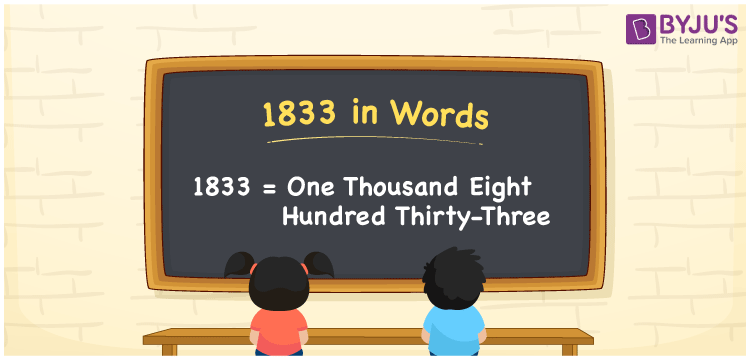# 1833 in Words

1833 in words can be written as One Thousand Eight Hundred Thirty-Three. If the water bill for a month is Rs. 1833, then you can say that “The water bill for a month is One Thousand Eight Hundred Thirty-Three Rupees”. Learn the place value of each digit in 1833 using the table provided for your reference. 1833 can be read as “One Thousand Eight Hundred Thirty-Three” in words.

 1833 in words One Thousand Eight Hundred Thirty-Three One Thousand Eight Hundred Thirty-Three in Numbers 1833

## 1833 in English Words## How to Write 1833 in Words?

Get to know how numbers can be written in words taking the place value chart provided below. The expanded form is also given here to improve conceptual knowledge among students.

 Thousands Hundreds Tens Ones 1 8 3 3

1833 in expanded form can be written as:

1 x Thousand + 8 × Hundred + 3 × Ten + 3 × One

= 1 x 1000 + 8 x 100 + 3 x 10 + 3 x 1

= 1000 + 800 + 30 + 3

= 1833

= One Thousand Eight Hundred Thirty-Three

Hence, 1833 in words is written as One Thousand Eight Hundred Thirty-Three.

1833 is a natural number that precedes 1834 and succeeds 1832.

1833 in words – One Thousand Eight Hundred Thirty-Three

Is 1833 an odd number? – Yes

Is 1833 an even number? – No

Is 1833 a perfect square number? – No

Is 1833 a perfect cube number? – No

Is 1833 a prime number? – No

Is 1833 a composite number? – Yes

## Frequently Asked Questions on 1833 in Words

Q1

### How to write 1833 in words?

1833 can be written in words as “One Thousand Eight Hundred Thirty-Three”.
Q2

### Write One Thousand Eight Hundred Thirty-Three in numbers.

One Thousand Eight Hundred Thirty-Three in numbers can be written as 1833.
Q3

### Is 1833 an even number?

No, 1833 is not an even number as it is not completely divisible by 2.